# 图文并茂！动态规划热门题目剖析

+关注继续查看

Doocs 开源社区的朋友们，你们好，我是 Chunel Feng。今天，我们就来简单分析几道动态规划（Dynamic Programming，简称 DP）相关的热门面试题目，陪着大家一起熟悉或复习一下这一部分知识。

•斐波那契数列

•一维相邻动态规划

•二维相邻动态规划

•间隔动态规划

## 一、爬楼梯

### 1. 题目链接：爬楼梯

假设你正在爬楼梯。需要 n 阶你才能到达楼顶。每次你可以爬 1 或 2 个台阶。你有多少种不同的方法可以爬到楼顶呢？注意：给定 n 是一个正整数。示例 1：输入： 2输出： 2解释： 有两种方法可以爬到楼顶。1.  1 阶 + 1 阶2.  2 阶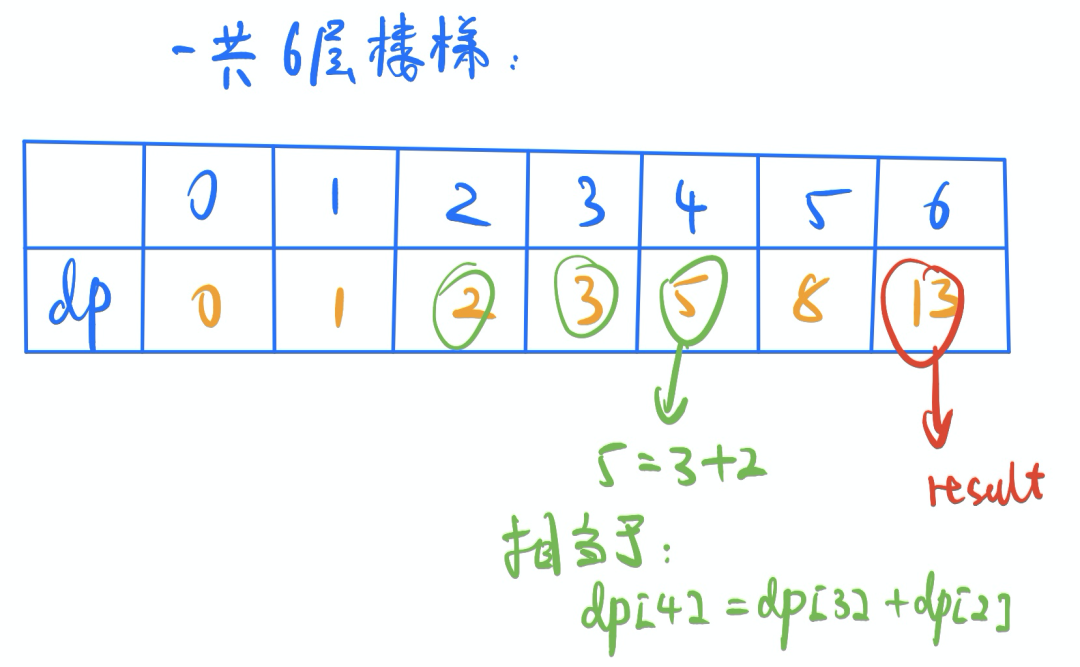### 2. 解题思路

这是一个很典型的斐波那契数列问题，也是一个很适合dp入门的题目。爬到3楼，只有两种可能：先爬到1楼，然后再一步上两层；或者先爬到2楼，然后再一步上一层。故：爬到3楼的方法数，等于爬到【1楼】的方法数和爬到【2楼】的方法数之和。爬到4楼的方法数，等于爬到【2楼】的方法数和爬到【3楼】的方法数之和。从而很容易推到出： dp[i] = dp[i-1] + dp[i-2]

### 3. 代码实现

class Solution {public:    int climbStairs(int n) {        // 不考虑n小于2的临界值情况了        vector<int> dp(n+1);        dp = 0;        dp = 1;        dp = 2;        for (int i = 3; i <= n; i++) {            dp[i] = dp[i - 1] + dp[i - 2];        }        return dp[n];    }};

## 二、连续子数组的最大和

### 1. 题目链接：连续子数组的最大和

给定一个整数数组 nums ，找到一个具有最大和的连续子数组（子数组最少包含一个元素），返回其最大和。示例 1：输入：nums = [-2,1,-3,4,-1,2,1,-5,4]输出：6解释：连续子数组 [4,-1,2,1] 的和最大，为 6 。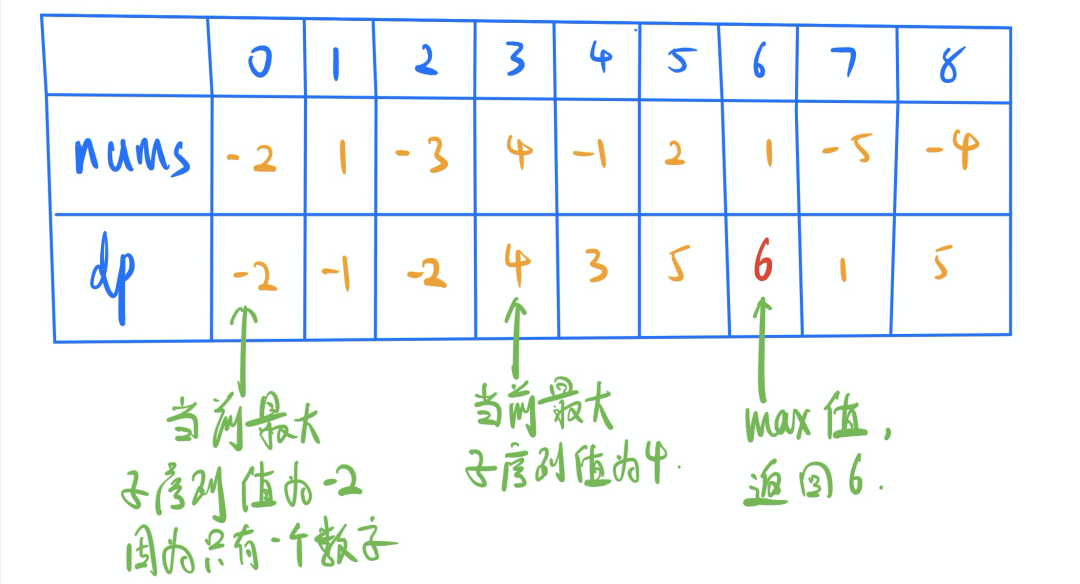### 2. 解题思路

这一题更准确的说，应该是一个贪心的题目。在这里，用一维dp的方法来实现。也是在一定程度上，说明一维dp和贪心之间，有一定的关联关系。解题思路，主要就是设定一个一维dp数组，数组的每一位记录当前最大的子序列值。体现在图中，就是dp = max(4, -2+4) = 4;dp = max(5, 1+5) = 6;dp[i] = std::max(dp[i-1] + nums[i], nums[i]);如果有超过之前最大值的，则更新result值。

### 3. 代码实现

class Solution {public:    int maxSubArray(vector<int>& nums) {        vector<int> dp(nums.size());        dp = nums;        int result = dp;        for (int i = 1; i < nums.size(); i++) {            dp[i] = std::max(dp[i-1] + nums[i], nums[i]);            result = std::max(dp[i], result);        }        return result;    }};

### 4. 补充说明

以上给出的解法，更偏向贪心和动态规划的结合。还有一种dp方法，是在dp数组中的每个值，均记录当前为止最大的子序列和是多少，然后返回dp[len-1]即可。

## 三、礼物的最大价值

### 1. 题目链接：礼物的最大价值

在一个 m*n 的棋盘的每一格都放有一个礼物，每个礼物都有一定的价值（价值大于 0）。你可以从棋盘的左上角开始拿格子里的礼物，并每次向右或者向下移动一格、直到到达棋盘的右下角。给定一个棋盘及其上面的礼物的价值，请计算你最多能拿到多少价值的礼物？示例 1:输入:[  [1,3,1],  [1,5,1],  [4,2,1]]输出: 12解释: 路径 1→3→5→2→1 可以拿到最多价值的礼物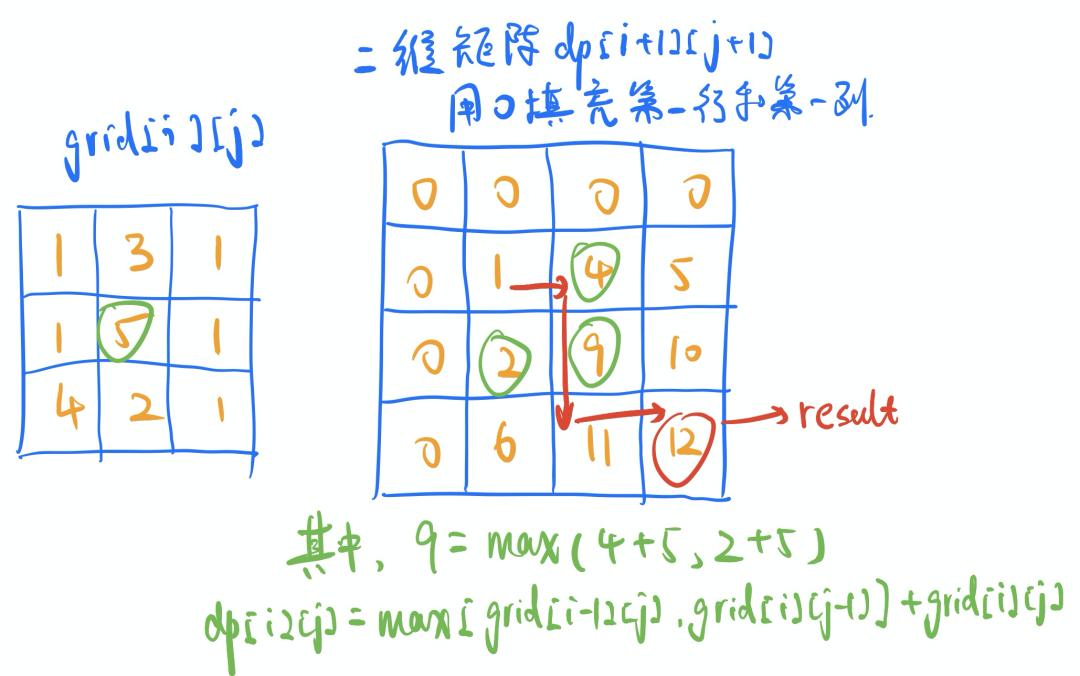### 2. 解题思路

这是一道典型的二维动态规划的题目。dp[i][j]值表示的表示的就是，走到当前位置，最多可以拿到的礼物价值之和。在计算dp[i][j]的过程中，该值总是与grid[i-1][j-1]、dp[i-1][j]、dp[i][j-1]相关。dp = max(dp, dp) + grid;dp = max(dp, dp) + grid;dp[i][j] = max(dp[i-1][j], dp[i][j-1]) + grid[i-1][j-1];体现在图中，就是 9 = max(4+5, 2+5)。依次计算下去，dp矩阵右下角的值（12），即为所求。而红色的箭头，表示的就是前进的方向。

### 3. 代码实现

class Solution {public:    int maxValue(vector<vector<int>>& grid) {        int h = grid.size();    // 行数        int w = grid.size();    // 列数        if (h == 0 || w == 0) {            return 0;        }        // 生成一个二维的dp数组        vector<vector<int>> dp(h+1, vector<int>(w+1, 0));        for (int i = 0; i < h+1; i++) {            for (int j = 0; j < w+1; j++) {                if (i != 0 && j != 0) {                    // 第0行和第0列值均为0，不参与计算。                    dp[i][j] = max(dp[i-1][j], dp[i][j-1]) + grid[i-1][j-1];                }            }        }        return dp[h][w];    }};

### 4. 补充说明

需要说明的是，这种解法相对比较容易理解，但在空间复杂度上有一定的优化空间。当计算dp[i][j]的值的时候，其实仅用到了i行和i-1行的数据。故计算的时候，可以仅保留上一行数据，甚至是上一行中涉及到的个别数据。具体方法，大家可以自己百度一下，当做一个知识点扩展了吧。

### 5. 相似推荐

类似的问题，给大家推荐 leetcode中【72.编辑距离】。需要说明的一点是，编辑距离的计算过程中，还有一些可以减少时间复杂度的彩蛋哦，大家自己去发掘哈。

## 四、零钱兑换

### 1. 题目链接：零钱兑换

给定不同面额的硬币 coins 和一个总金额 amount。编写一个函数来计算可以凑成总金额所需的最少的硬币个数。如果没有任何一种硬币组合能组成总金额，返回 -1。你可以认为每种硬币的数量是无限的。示例 1：输入：coins = [1, 2, 5], amount = 11输出：3解释：11 = 5 + 5 + 1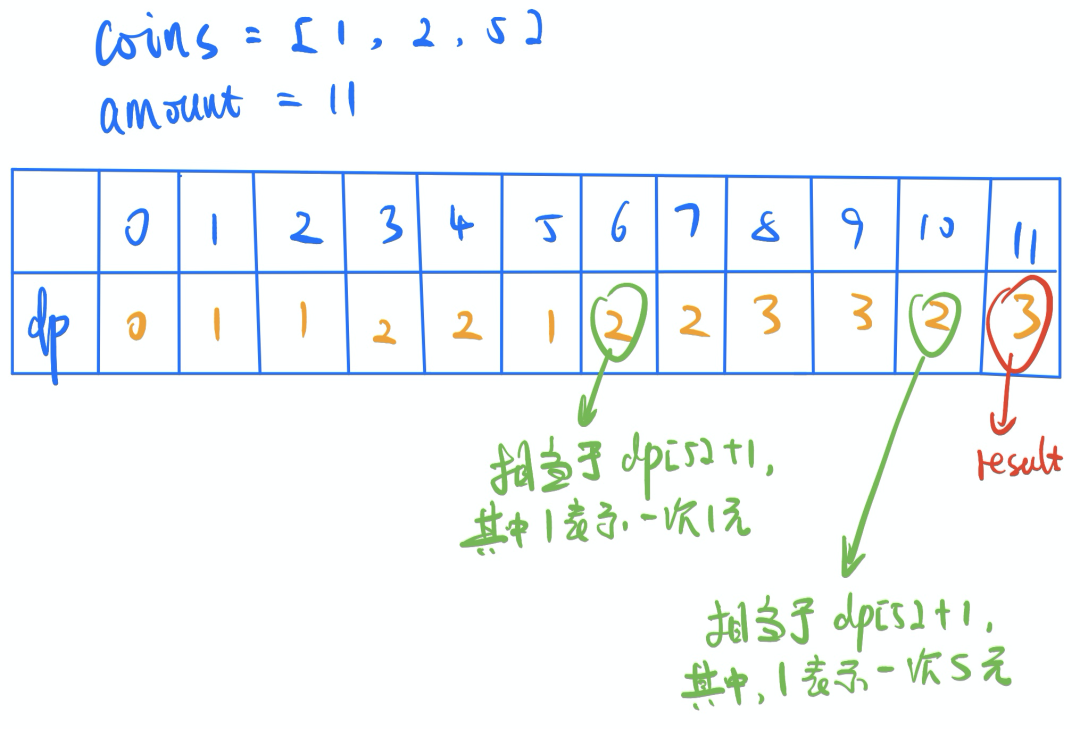### 2. 解题思路

找零问题，也是属于比较经典的一种 dp 问题。相比于上面几道题目，这一题的不同点在于，这里的 dp[i] 并不是与自己相邻的 dp[i-1] 产生关系。需要根据零钱的面额，向前找k个值（体现在 dp[i] = dp[i-coins[j]] + 1 这一句代码里）。

### 3. 代码实现

class Solution {public:    int coinChange(vector<int>& coins, int amount) {        vector<int> dp(amount+1, -1);    // 所有数据，默认用-1占位，表示没有被计算过        dp = 0;        for (int i = 1; i <= amount; i++) {            for (int j = 0; j < coins.size(); j++) {                /* 如果当前面值小于i，并且i-coins[j]面额的钱也可以被拼出。                   比如dp和2块钱面额，并且 dp[7-2] != -1 */                if (coins[j] <= i && dp[i-coins[j]] != -1) {                    /* 内部循环，如果dp[i]未被计算过，或者出现更低的值，则更新dp[i]的值 */                    if (dp[i] == -1 || dp[i] > dp[i-coins[j]] + 1) {                        dp[i] = dp[i-coins[j]] + 1;                    }                }            }        }        return dp[amount];    }};

### 4. 补充说明

上面是的解法，如果提前将coins中的值进行排序，还可以进行一些剪枝逻辑。比如，当一旦发现 coins[j]>i，则结束内部的循环。这个主要是为了说明，动态规划中合理的加入剪枝方法，可以一定程度的降低计算的时间复杂度。

### 5. 相似推荐

类似的问题，给大家推荐：背包问题。背包问题，更像是找零问题的二维版本，加入了重量和价值两个影响因素。具体问题就不给大家罗列了，自行百度一下或者去B站上搜，会有很多。记得要看哦。

## 引用链接

 一面之猿网: http://www.chunel.cn

 Caiss 智能搜索引擎: https://github.com/ChunelFeng/caiss

 Chunel Feng: https://github.com/ChunelFeng

 爬楼梯: https://leetcode-cn.com/problems/climbing-stairs/

 连续子数组的最大和: https://leetcode-cn.com/problems/lian-xu-zi-shu-zu-de-zui-da-he-lcof/

 礼物的最大价值: https://leetcode-cn.com/problems/li-wu-de-zui-da-jie-zhi-lcof/

 零钱兑换: https://leetcode-cn.com/problems/coin-change/19985 029364 064070 022618 0Xshell使用SSH远程登录阿里云ECS服务器CentOS7
22432 0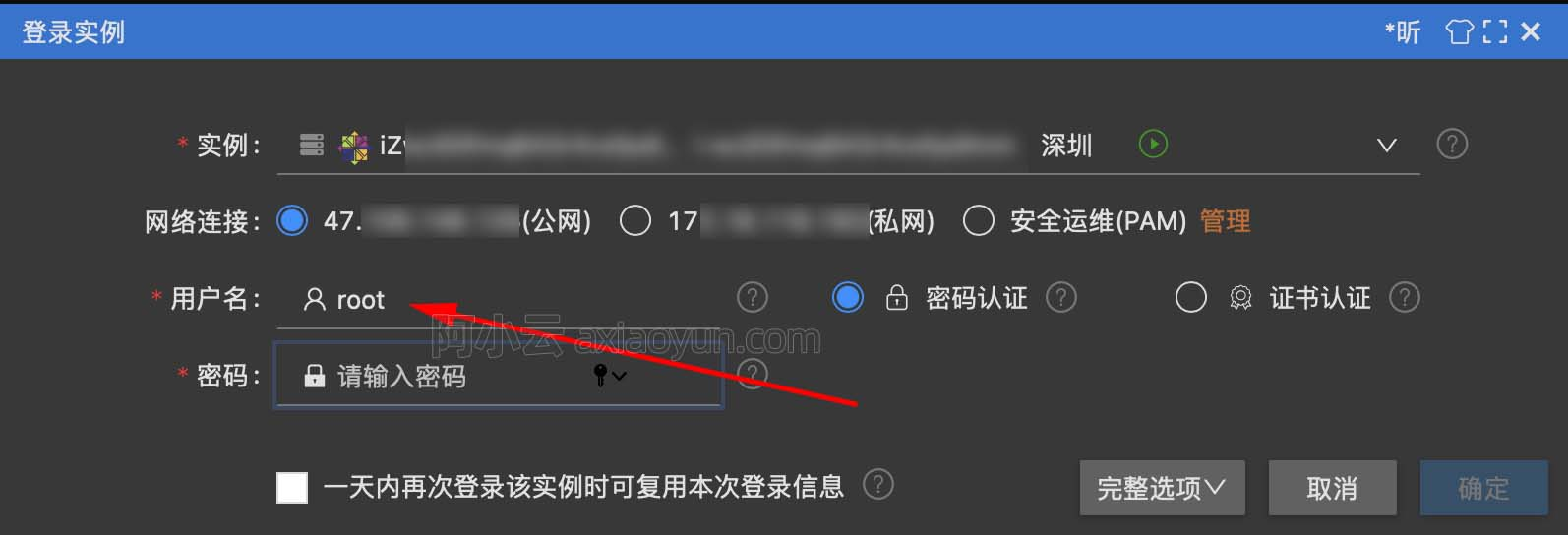16609 020823 015973 014909 023607 0
73

0

JS零基础入门教程（上册）